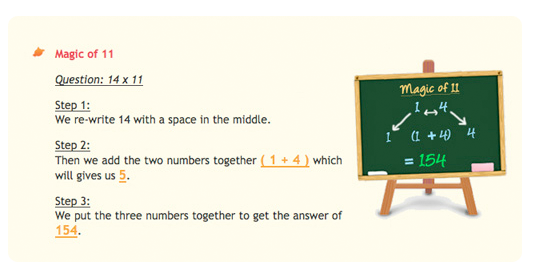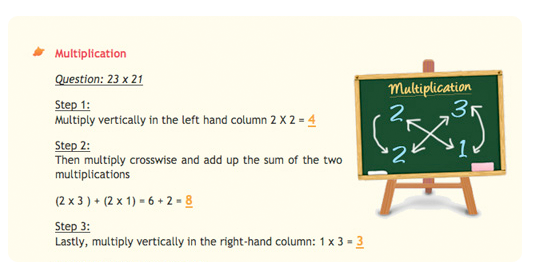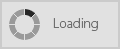6635 6395
Tutorials

The Magic of Vedic Math

Math Monkey's curriculum is primarily based on the mathematical techniques of Vedic Mathematics. Vedic Mathematics is the collective name given to a set of sixteen sutras (formulas) from the Vedic period in India from 1500 to 800 B.C. These sutras were originally written in Sanskrit and are built on the foundations of rigorous logic, thereby helping to guide and hone the human mind into approaches of logical reasoning. By learning these sutras, students will get to know the various ways of solving a math problem mentally and quickly.

The sixteen sutras of Vedic Math are given in word form such as "By one more than the one before" or "Vertically and Crosswise". They describe ways of using the mind, thereby giving direction to the mind, enabling the mind to solve algebra, geometry, calculus and other complex mathematical problems. Vedic Math concepts are simple to apply and as a result, answers can be obtained in one line or even mentally, thereby greatly expanding the thinking ability of the mind.

The advantages of Vedic Math are immense and these are:
• Through mental work, mental agility improves and the capacity to hold ideas in the mind increases.
• Mental work also improves concentration and memory skills.
• Rote learning of the multiplications tables are dispensed with.
• It enables faster calculations and enables the students to use it as a check and balance tool to the conventional method.
• The use of mental work reduces the need for fingers counting.
• It is easy to learn as the techniques are simple, direct and easy to remember.
• The focus on patterns and relationships in numbers help students to understand Math concepts better and faster.
• Vedic Math is extremely coherent and unified, hence it encourages innovation among the better students while its simplicity enables the weaker students to understand the basic concepts of Mathematics.Vedic Math Tutorial